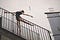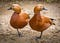# Steps for Linear Regression Algorithm (Simplified)

7 Important steps to keep in mind for creating a good linear regression modelPhoto by Liel Anapolsky on Unsplash

What is Linear Regression ?

Linear regression is a machine learning algorithm which estimates how a model is following a linear relationship between one response variable (denoted by y) and one or more explanatory variables (denoted by X1, X2, X3…. Xn). The response variable will dependent on how the explanatory variables changes and not the other way round. Response variable is also known as target or dependent variable while the explanatory variable is known as independent or predictor variables.

# Why your Linear Regression model would love some interpolation?

Interpolation or Extrapolation: Which one to choose and why ?

What is Interpolation:

It is a statistical method by which you can estimate unknown values by interpreting the trend followed by related known values, given that these unknown values must be present somewhere between the upper and lower limit of your observed dataset.

What is Extrapolation:

It is a statistical method by which you can estimate unknown values by interpreting the trend followed by related known values, given that these unknown values must be present outside the realm of upper and lower limit of your observed dataset.

Why Interpolation is…

# Death by Multicollinearity

## How dealing with predictor variables can save the life of your MLR ModelPhoto by Amir-abbas Abdolali on Unsplash

First, we have to understand what MLR (short for multiple linear regression) is, to fully grasp the concept of multicollinearity.

MLR estimates the relationship between one response variable (a.k.a., dependent variable) with two or more predictor variables (a.k.a., independent variable). For e.g., Profit is a dependent variable and is completely dependent on independent variables like Product quantity, cost of goods sold, admin expenses etc., and it is never other way around. The profit will linearly dependent on all these independent variables. We will see a real life example of MLR as we go through the post which shows how bmi…